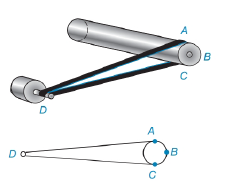Chapter 6.3, Problem 33E### Elementary Geometry for College St...

6th Edition
Daniel C. Alexander + 1 other
ISBN: 9781285195698

#### Solutions

Chapter
Section### Elementary Geometry for College St...

6th Edition
Daniel C. Alexander + 1 other
ISBN: 9781285195698
Textbook Problem
1 views

# The cylindrical brush on a vacuum cleaner is powered by an electric motor. In the figure, the drive shaft is at point D. if m A C ^ = 160 ° , find the measure of the angle formed by the drive belt at point D; that is find m ∠ DTo determine

To find:

To find mD.

Explanation

Given that, mAC^=160°.

From the diagrammatic representation,

Let D is vertex outside the circle therefore,

mD=mABC^-mAC^2

Since mABC^=360°-mAC^

Substitute mABC^=360°-mAC^ in the above equation to get he following,

mD=360°-mAC^-mAC^2

mD=

### Still sussing out bartleby?

Check out a sample textbook solution.

See a sample solution

#### The Solution to Your Study Problems

Bartleby provides explanations to thousands of textbook problems written by our experts, many with advanced degrees!

Get Started

#### Find more solutions based on key concepts